# How to denote subset(⊂) in LaTeX?

In this tutorial, we will cover how to denote a subset using Latex.

With subsets we use non subset, super subset and non super subset. And it is very important for you to know how to represent these symbols with the help of latex.

So, take a look at this table below. Here is the list of latex commands.

 Set Tearm LaTeX Subset \subseteq Non Subset \nsubsteq Proper Subset \subset Non Proper Subset \not \subset

## Subset and non subset symbol in LaTeX

Mathematically, you need to use the symbol to represent the subset.

 Properties Value Symbol Subset Argument No Command \subseteq Example \subseteq →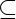You need to use the \subseteq command to identify subset symbols without installing any packages using Latex.

\documentclass{article}
\begin{document}
$a \subseteq b$
\end{document}

Output :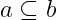Second, you need to use the \nsubseteq command for non-subset symbols.

 Properties Value Symbol Non Subset Argument No Command \nsubseteq Example \nsubseteq →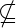Suppose two sets a and b are taken here. And the number of elements of a is less than b and unequal. Then

\documentclass{article}
\begin{document}
$a \nsubseteq b$
\end{document}


Output :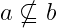Each set is its own subset

\documentclass{article}
\begin{document}
$P \subseteq P$
\end{document}


Output :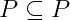## Proper subset and non proper subset symbol in LaTeX

Proper subset is not a separate concept, it is a part of subset. In latex, the proper subset symbol is represented by the ⊂ symbol.

\documentclass{article}
\begin{document}
$a \subset b$
\end{document}


Output :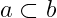If you look at the program above, you will understand that the \subset command has been used for the proper subset symbol.

In the same way, for not proper subset you need to use both \not and \subset commands together.

\documentclass{article}
\begin{document}
$a \not \subseteq b$
\end{document}


Output :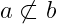## Latex subset with not equal symbol

Suppose, p is a subset of q but p is not equal to q. In that case, unequal symbols are used with subset symbols. For example, take a look at the following program.

\documentclass{article}
\usepackage{amssymb}
\begin{document}
$$P \subsetneq Q$$
$$P \subsetneqq Q$$
\end{document}

Output :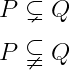In this case, you need to use the amssymb package. And before the document starts, you need to define the package and use the \usepackage command.